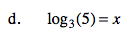Home > CCA2 > Chapter 8 > Lesson 8.3.2 > Problem8-158

8-158.
1. Solve each equation. Homework Help ✎

1. log3(2x − 1) = −2

2. 5log5(x) = 3

3. log2(x) − log2(3) = 4

4. log3(5) = x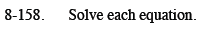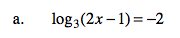Convert the equation into exponential form.
3−2 = 2x − 1

Solve for x.

$x = \frac{5}{9}$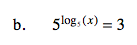Remember the Inverse Relationship of Logarithms:

$b^{ \log_b (N)}$

x = 3

$\text{log}(N)-\text{log}(M)= \text{log} \left( \frac{N}{M} \right)$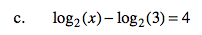Remember the Quotient Property of Logarithms:

$3^x = 5$